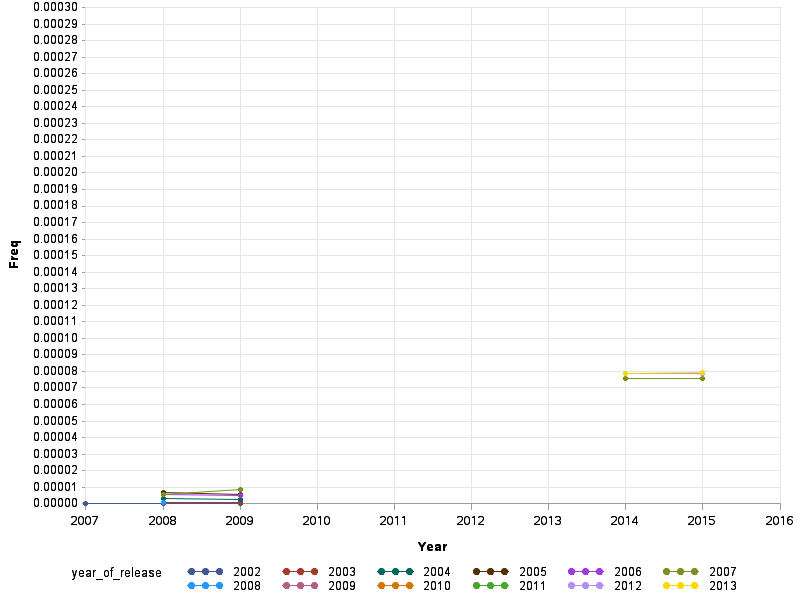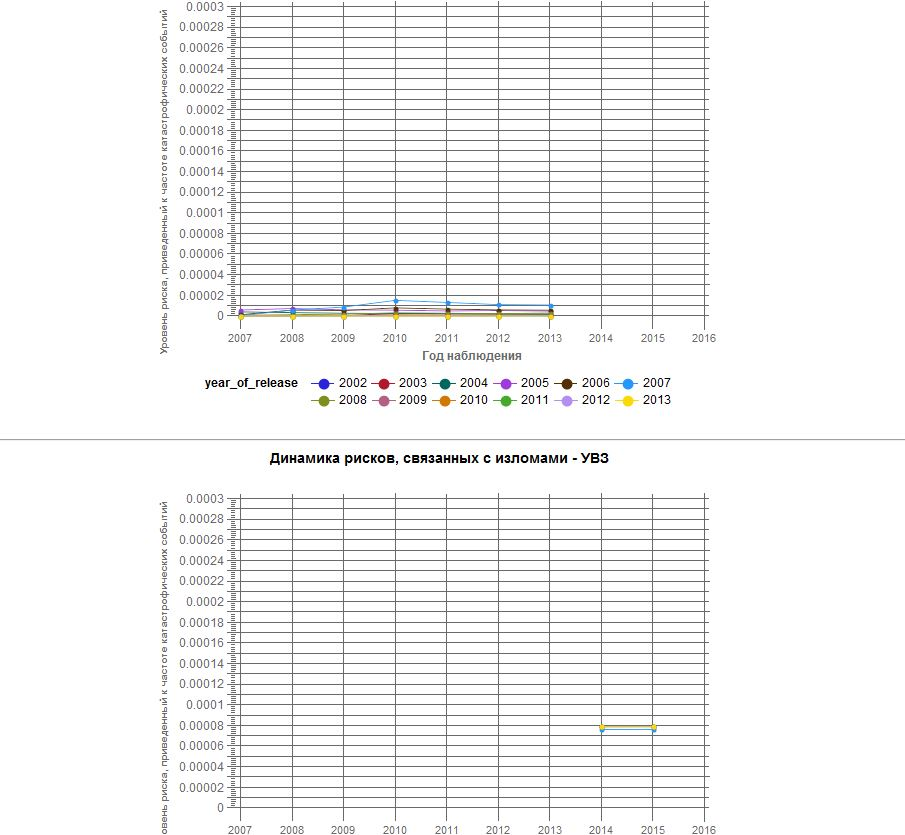## Overlay option with GPLOT

Good day for all!

Sorry, I have a new problem with GPLOT.

I'm trying to draw two parts of graph using "overlay" option.

I have many dots in the first part and the same number dots in the other one.

The both part must be on one graph, but the system draws for me two different graphs for each part separatly.

In first part I use 'year'  (ORDER = (2007 to 2016 by 1)) for x, 'freq3' (ORDER = (0 to 0.0003 by 0.00001)) for y. And 'year_of_release' for groupping (number like 2007).

In second part I use the same 'year' for x, 'year_of_release' for groupping and 'freq3_pr' (absolute identical to freq3 order). I don't understand where is the problem.

The second part of my graph is a forecast for values from first part. I thought about renaming 'freq3_pr' to 'freq' and working with data like one block but I need to draw the line of forecast in graph like dotted line and other part of graph - like a straight line. Maybe my problem has other answer?

There is my code:

goptions

RESET = all

DEVICE = activex

;

symbol1

INTERPOL = join

VALUE = dot

LINE = 1

WIDTH = 1

HEIGHT = 0.7

;

symbol2

INTERPOL = join

VALUE = dot

LINE = 1

WIDTH =3

HEIGHT = 2

;

Axis1

LABEL = (font="Arial/bold" a=90    Justify=center "Freq")

ORDER = (0 to 0.0003 by 0.00001)

OFFSET = (0,0)

;

Axis2

ORDER = (2007 to 2016 by 1)

LABEL = (font="Arial/bold"    Justify=center "Year")

VALUE = (font="Arial" HEIGHT=9pt )

MINOR = none

OFFSET = (0,0)

;

PROC GPLOT DATA = mergeDots ;

PLOT freq3 * year  = year_of_release freq3_pr * year  = year_of_release / OVERLAY

VAXIS = AXIS1

HAXIS = AXIS2

ANNOTATE = labelForAnnotable

NOFRAME

GRID

;

RUN;

1 ACCEPTED SOLUTION

Accepted Solutions

## Re: Overlay option with GPLOT

One way would be to generate the plots separately, and then combine them using Proc Greplay.

(but I would recommend re-evaluating what it is you really want to show on the plot, and maybe

try a different approach altogether).

data mergeDots;

informat freq3 freq3_pr best12.;

input freq3 freq3_pr year year_of_release;

datalines;

1.54E-7         .       2007    2002

1.23E-7         .       2008    2002

6.15E-7         .       2008    2003

3.08E-6         .       2008    2004

7.06E-6         .       2008    2005

5.57E-6         .       2008    2006

5.57E-6         .       2008    2007

1.07E-6         .       2008    2008

1.02E-7         .       2009    2002

5.12E-7         .       2009    2003

2.56E-6         .       2009    2004

5.8E-6          .       2009    2005

5.11E-6         .       2009    2006

8.49E-6         .       2009    2007

.       0.0000759554    2014    2007

.       0.0000786768    2014    2008

.       0.0000786678    2014    2009

.       0.000078661     2014    2010

.       0.0000789464    2014    2011

.       0.0000789044    2014    2012

.       0.0000790309    2014    2013

.       0.0000759931    2015    2007

.       0.0000787159    2015    2008

.       0.0000787068    2015    2009

.       0.0000787       2015    2010

.       0.0000789856    2015    2011

.       0.0000789435    2015    2012

.       0.0000790702    2015    2013

;

run;

goptions RESET=all DEVICE=png;

Axis1 LABEL=(font="Albany amt/bold" a=90 Justify=center "Freq")
ORDER=(0 to 0.0003 by 0.00001) OFFSET=(0,0);

Axis2 ORDER=(2007 to 2016 by 1)
LABEL=(font="Albany amt/bold" Justify=center "Year")
VALUE=(font="Albany amt" HEIGHT=9pt )
MINOR=none OFFSET=(0,0);

symbol INTERPOL=join VALUE=dot LINE=1 WIDTH=1 HEIGHT=0.7;

%macro delcat(catname);
%if %sysfunc(cexist(&catname)) %then %do;
proc greplay nofs igout=&catname;
delete _all_;
run;
%end;
quit;
%mend delcat;
%delcat(work.gseg);

PROC GPLOT DATA=mergeDots;
PLOT freq3*year=year_of_release /
VAXIS=AXIS1 HAXIS=AXIS2
NOFRAME GRID name='out1';
RUN;

PROC GPLOT DATA=mergeDots;
PLOT freq3_pr*year=year_of_release /
VAXIS=AXIS1 HAXIS=AXIS2
NOFRAME GRID name='out2';
RUN;

proc greplay tc=tempcat nofs igout=work.gseg;

tdef whole des='whole screen'

1/llx = 0   lly =  0

ulx = 0   uly =100

urx =100  ury =100

lrx =100  lry =  0

;

template = whole;

treplay 1:out1 1:out2;

run;7 REPLIES 7

## Re: Overlay option with GPLOT

Some example data that duplicates the problem would help.

## Re: Overlay option with GPLOT

 freq3 freq3_pr year year_of_release 0.0000001540 . 2007 2002 0.0000007680 . 2007 2003 0.0000038500 . 2007 2004 0.0000056200 . 2007 2005 0.0000011200 . 2007 2006 0.0000001540 . 2007 2007 0.0000002100 . 2008 2002 0.0000000000 . 2008 2003 0.0000000000 . 2008 2004 0.0000000000 . 2008 2005 . 0.0002387854 2014 2002 . 0.000240714 2014 2003 . 0.0002407216 2014 2004 . 0.0002407423 2014 2005 . 0.0002410621 2014 2006 . 0.0002410372 2014 2007

## Re: Overlay option with GPLOT

I don't think your data table came through cleanly (or maybe they're using a format I'm not familiar with).

Your numbers appear to have 2 decimals, and a space in them, such as ... freq3="1. 0.0000001540".

If I chop the '1. ' off, what's left (such as 0.0000001540) is still not in the AXIS1 range you specified (0 to 0.0003).

## Re: Overlay option with GPLOT

Thank you so much!

## Re: Overlay option with GPLOT

I've corrected the data table. Now it's like this:

 Freq3 Freq3_pr year Year_of_release 1.54E-7 . 2007 2002 1.23E-7 . 2008 20023 6.15E-7 . 2008 2003 3.08E-6 . 2008 2004 7.06E-6 . 2008 2005 5.57E-6 . 2008 2006 5.57E-6 . 2008 2007 1.07E-6 . 2008 2008 1.02E-7 . 2009 2002 5.12E-7 . 2009 2003 2.56E-6 . 2009 2004 5.8E-6 . 2009 2005 5.11E-6 . 2009 2006 8.49E-6 . 2009 2007 . 0.0000759554 2014 2007 . 0.0000786768 2014 2008 . 0.0000786678 2014 2009 . 0.000078661 2014 2010 . 0.0000789464 2014 2011 . 0.0000789044 2014 2012 . 0.0000790309 2014 2013 . 0.0000759931 2015 2007 . 0.0000787159 2015 2008 . 0.0000787068 2015 2009 . 0.0000787 2015 2010 . 0.0000789856 2015 2011 . 0.0000789435 2015 2012 . 0.0000790702 2015 2013

Format BEST12 maybe it's important. There is a picture of my graphs:They still be separately.

## Re: Overlay option with GPLOT

One way would be to generate the plots separately, and then combine them using Proc Greplay.

(but I would recommend re-evaluating what it is you really want to show on the plot, and maybe

try a different approach altogether).

data mergeDots;

informat freq3 freq3_pr best12.;

input freq3 freq3_pr year year_of_release;

datalines;

1.54E-7         .       2007    2002

1.23E-7         .       2008    2002

6.15E-7         .       2008    2003

3.08E-6         .       2008    2004

7.06E-6         .       2008    2005

5.57E-6         .       2008    2006

5.57E-6         .       2008    2007

1.07E-6         .       2008    2008

1.02E-7         .       2009    2002

5.12E-7         .       2009    2003

2.56E-6         .       2009    2004

5.8E-6          .       2009    2005

5.11E-6         .       2009    2006

8.49E-6         .       2009    2007

.       0.0000759554    2014    2007

.       0.0000786768    2014    2008

.       0.0000786678    2014    2009

.       0.000078661     2014    2010

.       0.0000789464    2014    2011

.       0.0000789044    2014    2012

.       0.0000790309    2014    2013

.       0.0000759931    2015    2007

.       0.0000787159    2015    2008

.       0.0000787068    2015    2009

.       0.0000787       2015    2010

.       0.0000789856    2015    2011

.       0.0000789435    2015    2012

.       0.0000790702    2015    2013

;

run;

goptions RESET=all DEVICE=png;

Axis1 LABEL=(font="Albany amt/bold" a=90 Justify=center "Freq")
ORDER=(0 to 0.0003 by 0.00001) OFFSET=(0,0);

Axis2 ORDER=(2007 to 2016 by 1)
LABEL=(font="Albany amt/bold" Justify=center "Year")
VALUE=(font="Albany amt" HEIGHT=9pt )
MINOR=none OFFSET=(0,0);

symbol INTERPOL=join VALUE=dot LINE=1 WIDTH=1 HEIGHT=0.7;

%macro delcat(catname);
%if %sysfunc(cexist(&catname)) %then %do;
proc greplay nofs igout=&catname;
delete _all_;
run;
%end;
quit;
%mend delcat;
%delcat(work.gseg);

PROC GPLOT DATA=mergeDots;
PLOT freq3*year=year_of_release /
VAXIS=AXIS1 HAXIS=AXIS2
NOFRAME GRID name='out1';
RUN;

PROC GPLOT DATA=mergeDots;
PLOT freq3_pr*year=year_of_release /
VAXIS=AXIS1 HAXIS=AXIS2
NOFRAME GRID name='out2';
RUN;

proc greplay tc=tempcat nofs igout=work.gseg;

tdef whole des='whole screen'

1/llx = 0   lly =  0

ulx = 0   uly =100

urx =100  ury =100

lrx =100  lry =  0

;

template = whole;

treplay 1:out1 1:out2;

run;## Re: Overlay option with GPLOT

Thank you again!

Why this method is not recomended by you? I thought about using %LINE for second part. Maybe this will be better? (I was confuse in position data for %LINE and stopped).

Discussion stats
• 7 replies
• 1450 views
• 3 likes
• 3 in conversation